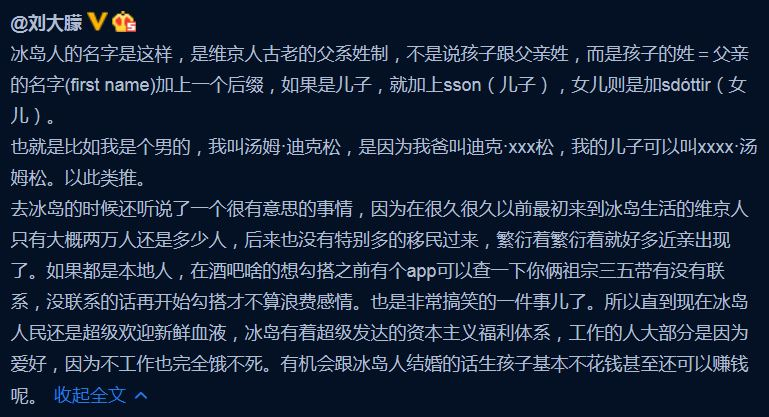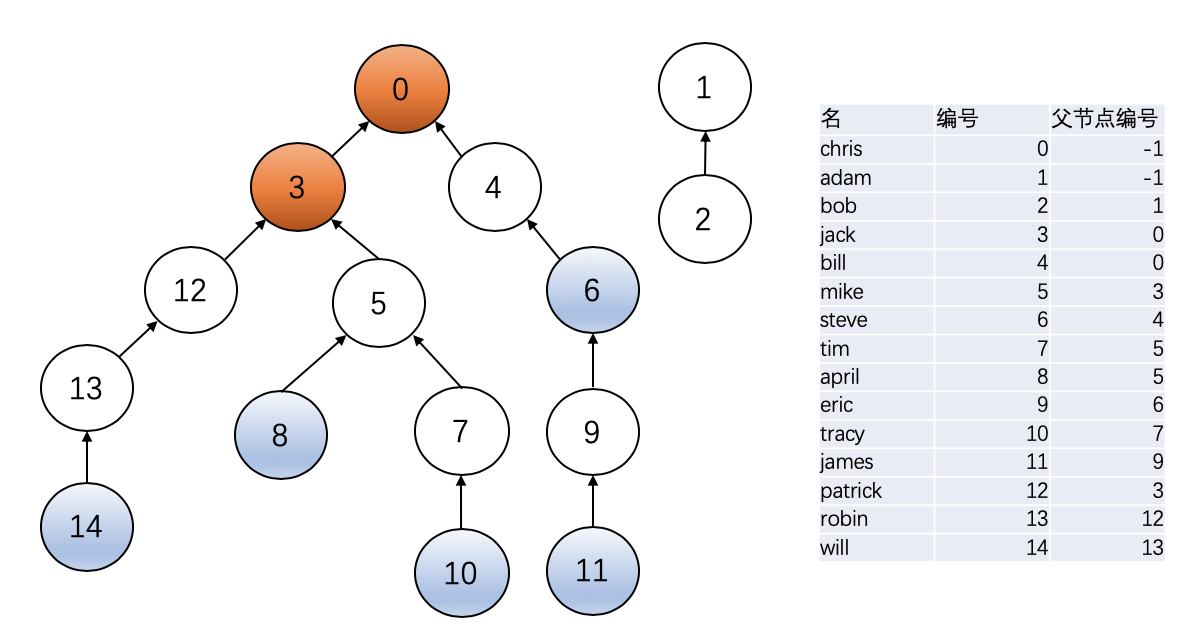# L2-030 冰岛人 （25 分)

##### L2-030 冰岛人 （25 分)

2018年世界杯，冰岛队因1:1平了强大的阿根廷队而一战成名。好事者发现冰岛人的名字后面似乎都有个“松”（son），于是有网友科普如下：##### 输入样例：

15
chris smithm
jack chrissson
bill chrissson
mike jacksson
steve billsson
tim mikesson
april mikesdottir
eric stevesson
tracy timsdottir
james ericsson
patrick jacksson
robin patricksson
will robinsson
6
tracy tim james eric
will robin tracy tim
april mike steve bill
tracy tim tracy tim
x man april mikes

Yes
No
No
Whatever
Whatever
NA

##### 题解##### 代码
#include <bits/stdc++.h>
using namespace std;
bool judge(vector<int> &F, int s1, int s2)
{
int n = F.size();
vector<int> count(n, 0);
vector<int> dist1(n, 0);
vector<int> dist2(n, 0);
int t ;
count[s1]++;
count[s2]++;
while(F[s1] != -1)
{
t = F[s1];
count[t]++;
dist1[t] = dist1[s1] + 1;
if(t == s2)			//直系祖宗...太乱了，直接false，不加这个，会有两个点过不去
return false;
s1 = t;
}
while(F[s2] != -1)
{
t = F[s2];
count[t]++;
dist2[t] = dist2[s2] + 1;
if(count[t] > 1)
{
if(dist2[t]>=4 && dist1[t] >= 4)
return true;
else
return false;
}
s2 = t;
}
return true;
}
int main()
{
int n;
cin >> n;
string f1, f2;
vector<bool> sex(n);
vector<vector<string> > record(n);
map<string, int> M;			//给每个人编号
vector<int> F(n, -1);		//父节点编号
int cnt = 0;
for(int i=0;i<n;i++)		//编号
{
cin >> f1 >> f2;
M.insert(make_pair(f1, cnt));
int l = f2.size();
if(f2[l-1] == 'm' || f2[l-1] == 'n')
sex[cnt] = 1;       //男
else
sex[cnt] = 0;
cnt++;
record[i].push_back(f1);
record[i].push_back(f2);
}
string par;
for(int i=0;i<n;i++)		//找父节点
{
f1 = record[i];
f2 = record[i];
int len = f2.size();
if(f2[len-1] != 'r' && f2[len-1] != 'n')	//老祖宗
continue;
if(sex[M[f1]] == true)
par = f2.substr(0, len-4);
else
par = f2.substr(0, len-7);
F[M[f1]] = M[par];
}
int m;
cin >> m;
string t1,t2;
for(int i=0;i<m;i++)
{
cin >> f1 >> f2 >> t1 >> t2;
if(M.find(f1) == M.end() || M.find(t1) == M.end() )
{
cout << "NA" << endl;
continue;
}
if(sex[M[f1]] == sex[M[t1]])
{
cout << "Whatever" << endl;
continue;
}
if(judge(F, M[f1], M[t1]))
{
cout << "Yes" << endl;
}
else
cout << "No" << endl;
}
return 0;
}

04-072112
11-1758
08-08353
01-02507
03-171306
01-26691
10-06695
06-05233
03-313924
10-19701
01-27848
03-06346
08-10728
11-293294
04-021335
04-11310
05-1761
04-041435
03-10753

### “相关推荐”对你有帮助么？

•非常没帮助
•没帮助
•一般
•有帮助
•非常有帮助被折叠的  条评论 为什么被折叠?到【灌水乐园】发言¥2 ¥4 ¥6 ¥10 ¥20余额支付 (余额：-- )扫码支付获取中扫码支付点击重新获取扫码支付1.余额是钱包充值的虚拟货币，按照1:1的比例进行支付金额的抵扣。
2.余额无法直接购买下载，可以购买VIP、C币套餐、付费专栏及课程。余额充值